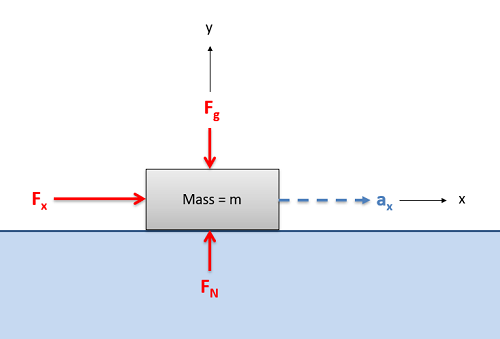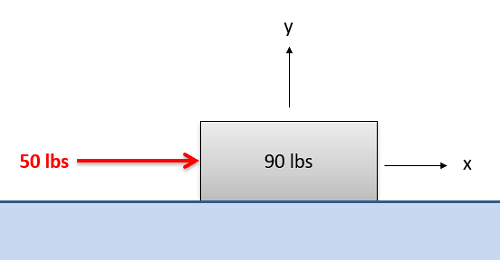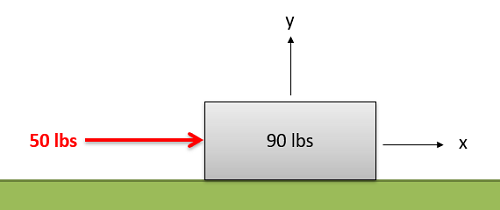﻿ Mechanics Map - The Equation of Motion in One Dimension

# The Equation of Motion in One Dimension

Kinetics is the branch of dynamics that deals with the relationship between motion and the forces that cause that motion. The basis for all of kinetics is Newton's Second Law, which relates forces and accelerations for a given body. In its basic form, Newton's Second Law states that the sum of the forces on a body will be equal to mass of that body times the rate of acceleration. For bodies in motion, we can write this relationship out as the equation of motion.

 $\sum \vec{F}=m*\vec{a}$

In cases where accelerations only exist in a single dimension, we can reduce the above vector equation into a single scalar equation. Calling that single direction the x direction, we arrive at the single equation of motion shown below. By entering known forces or accelerations, we can use this equation to solve for a single unknown force or acceleration term.This box being pushed along a frictionless surface can be examined as a one dimensional kinetics problem. Acceleration exists only in the x direction, related by the equation of motion to the single unbalanced force in the x direction. Because the forces in the y direction are balanced, the acceleration in that direction will be zero.
 $\sum F_{x}=m*a_{x}=m*\ddot{x}$

Kinetics and the equation(s) of motion relate forces and accelerations, and are often used in conjunction with the kinematics equations, which relate positions, velocities and accelerations as discussed in the previous chapter. Depending on the problem being examined, the kinematics equations may need to be examined either before or after the kinetics equations.

## Worked Problems:

### Question 1:

A block with a weight of 90 pounds sits on a frictionless surface as shown below. A 50 pound force is then applied in the x direction as shown below.

• What is the rate of acceleration of the block?
• What is the velocity and displacement three seconds after the force is applied?### Question 2:

A block with a weight of 90 pounds sits on a surface with a kinetic coefficient of friction of .2 as shown below. A 50 pound force is then applied in the x direction as shown below.

• What is the rate of acceleration of the block?
• What is the velocity and displacement three seconds after the force is applied?### Question 3:

A 2000 lb elevator decelerates from a speed of 25 ft/s downward to a stop in a distance of 50 ft.

• What is the average rate of deceleration?
• What is the tension in the cable supporting the elevator during this period?### Question 4:

A rocket test sled is being used to test a solid rocket booster (m=1000kg). It’s known that generally a solid rocket booster’s force will fit the equation F = A + Bt – Ct2. If the rocket has an initial thrust of 10 kN, and achieves a speed of 150 m/s and travels 700m during a 10 second test run, determine the constants A, B and C for the rocket.